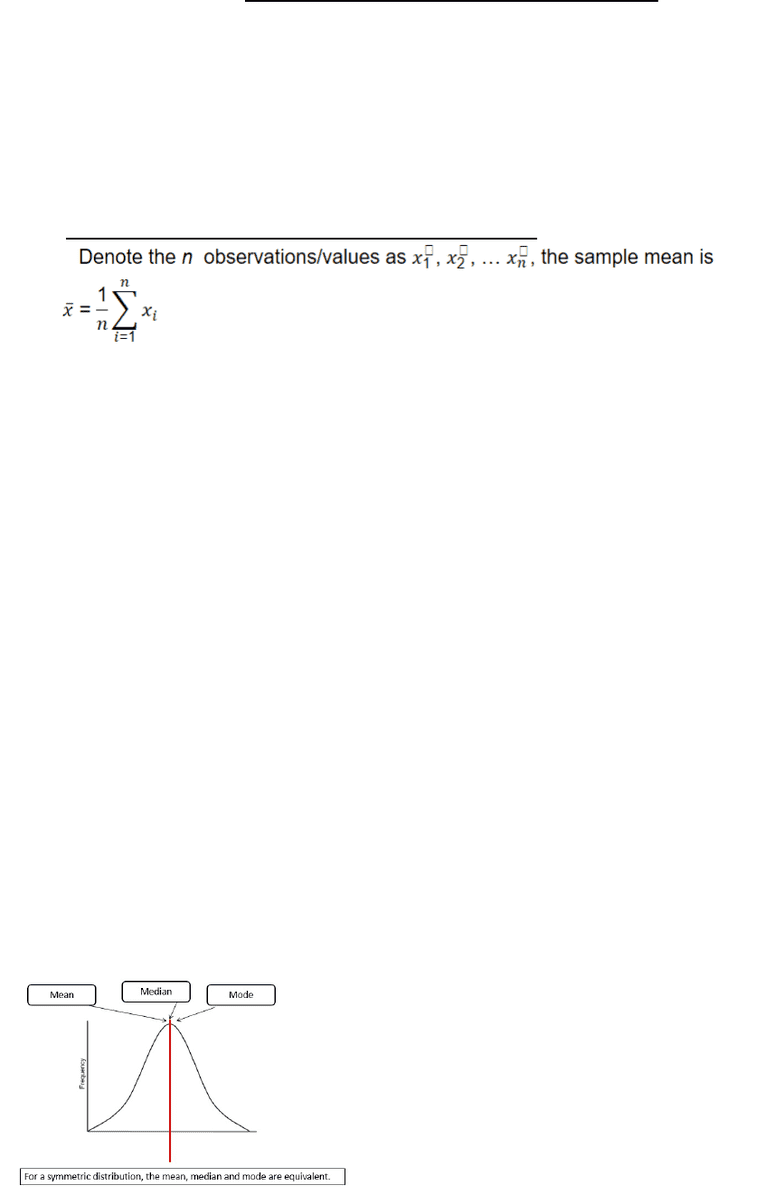Textbook Notes (270,000)
CA (160,000)
UW (5,000)
STAT (30)
STAT211 (20)
Chapter 3

STAT211 Chapter Notes - Chapter 3: 5,6,7,8, Quartile, Standard Deviation

Department
Statistics
Course Code
STAT211
Professor
Joslin Goh
Chapter
3

This preview shows page 1. to view the full 4 pages of the document.C3: Measures of Central Tendency
Centre of the Distribution
Three common ways to measure the center of the distribution are:
Mean
Median
Mode: The value that occurs most frequently
Mean
Usually it is the sample mean
Sample mean is not the same of the population mean
Median
The middle value with half of the data above it and half below it
The value that falls in the middle after all the values been arranged in ascending order
Assume that there are n observations
If n is odd, the median is the value in the middle
If n is even, the median is the average of the two values in the middle
Example: Odd amount of numbers
5, 5, 6, 7, 9
Mode: 5
Mean: 6.4
Median: 6
Example: Even amount of numbers
1, 5, 5, 6, 7, 8
Mode: 5
Mean: 5.5
Median: 5.5 (5+6)/2
Example: 2, 4, 7, 1, 2, 30, 1, 3
1, 1, 2, 2, 3, 4, 7, 30
Mode: 1, 2
Mean: 6.375
Median: 2.5
Mean, median, mode are only same/similar if there's a symmetric distribution